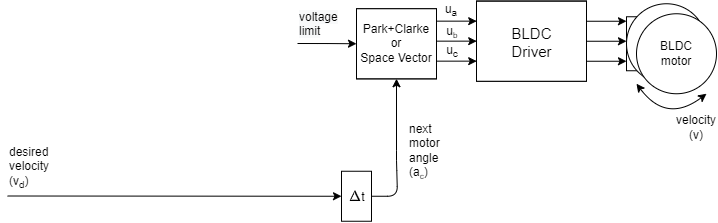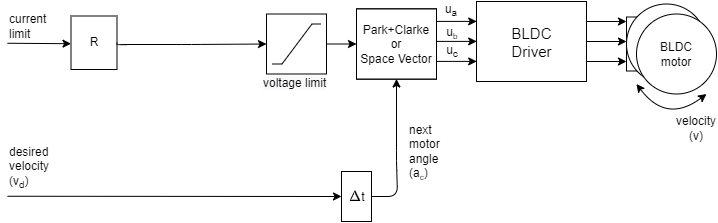# Velocity open-loop control

This control loop allows you to spin your BLDC motor with desired velocity without using position sensor. This mode is enabled by:

// set velocity control open-loop mode
motor.controller = MotionControlType::velocity_openloop;You can test this algorithm by running the examples in motion_control/openloop_motor_control/ folder.

This control algorithm is very simple. User can set the target velocity it wants to achieve vd, the algorithm is going to integrate it in time to find out what is the angle it needs to set to the motor ac in order to achieve it. Then the maximal allowed voltage motor.voltage_limit is going to be applied in the direction of the ac using SinePWM or SpaceVectorPWM modulation.

This is the simplified version of the calculation of the next angle to set to the motor:

next_angle = past_angle + target_velocity*d_time;


You need to know the target_velocity, sample time d_time and past value of the angle past_angle you have set to the motor.

## Configuration

There are only three main parameters of the velocity open-loop control

// choose FOC modulation (optional) - SinePWM or SpaceVectorPWM
motor.foc_modulation = FOCModulationType::SinePWM;

// limiting voltage
motor.voltage_limit = 3;   // Volts
// or current  - if phase resistance provided
motor.current_limit = 0.5 // Amps


The velocity open-loop control will (if not provided phase resistance) set the voltage to the motor equal to the motor.voltage_limit

voltage = voltage_limit; // Volts


This is very inefficient, as for different motors with different phase resistances the same voltage values can produce wildly different currents. For gimbal motor, you can run it in the open loop with the voltage limits of 5-10 Volts and it will reach the currents of 0.5-2 amps as it has the pahse resistance from 5-15 Ohms. For drone motors, the voltage limits should stay very low, under 1 volt. Because they have pahse resisatnce of 0.05 to 0.2 Ohms.

### Current limiting approaches

We suggest you to provide the motor class with the phase_resistance value and set the motor.current_limit instead the voltage limit. This current might be surpassed but at least you will know an approximate current your motor is drawing. You can calculate the current the motor is going to be producing by checking the motor resistance phase_resistance and evaluating:

voltage = current_limit * phase_resistance; // Amps


The best way to use this control strategy would be to provide both phase resistance value and KV rating of your motor. The the library would be able to calculate he back-EMF voltage and much more precisely estimate the consumed current. And with the current and the back-EMF current the library can set much more appropriate voltage to the motor.

voltage = current_limit*phase_resistance + desired_velocity/KV; // Amps


### Changing limits in real-time

Also, you can change the voltage/current limit in real-time if you need this kind of behavior in your application.

## Velocity open-loop control example

Here is one basic example of the velocity open-loop control with the complete configuration. The program will set the target velocity of 2 RAD/s and maintain it, and the user can change the target velocity using serial terminal.

// Open loop motor control example
#include <SimpleFOC.h>

// BLDC motor & driver instance
// BLDCMotor( pp number , phase resistance)
BLDCMotor motor = BLDCMotor(11 , 12.5, 100);
BLDCDriver3PWM driver = BLDCDriver3PWM(9, 5, 6, 8);

// instantiate the commander
Commander command = Commander(Serial);
void doTarget(char* cmd) { command.scalar(&motor.target, cmd); }
void doLimitCurrent(char* cmd) { command.scalar(&motor.current_limit, cmd); }

void setup() {

// driver config
// power supply voltage [V]
driver.voltage_power_supply = 12;
driver.init();
// link the motor and the driver

// limiting motor current (provided resistance)
motor.current_limit = 0.5;   // [Amps]

// open loop control config
motor.controller = MotionControlType::velocity_openloop;

// init motor hardware
motor.init();
motor.initFOC();

Serial.begin(115200);
_delay(1000);
}

void loop() {
motor.loopFOC();
// open loop velocity movement
// using motor.current_limit and motor.velocity_limit
motor.move();

// user communication
command.run();
}